# Introducing Current Electricity Introducing Current Electricity the controlled

• Slides: 28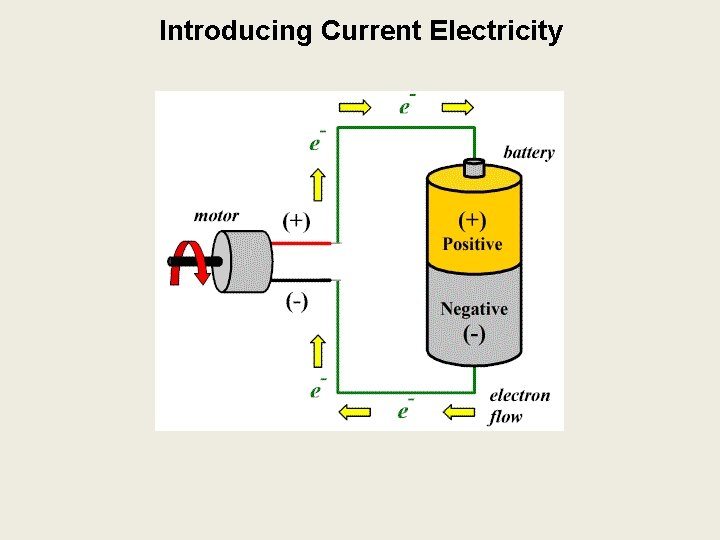Introducing Current Electricity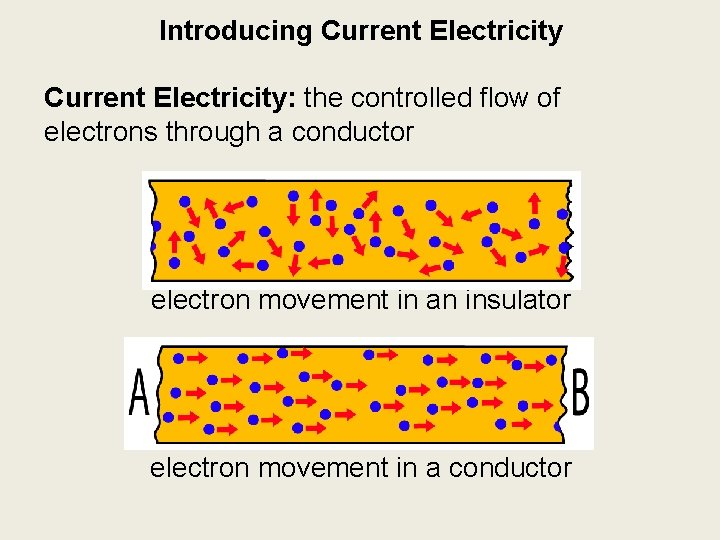Introducing Current Electricity: the controlled flow of electrons through a conductor electron movement in an insulator electron movement in a conductor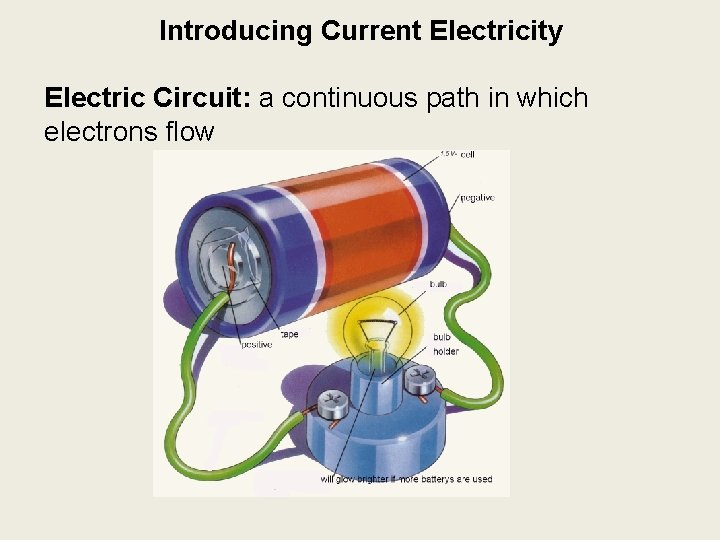Introducing Current Electricity Electric Circuit: a continuous path in which electrons flow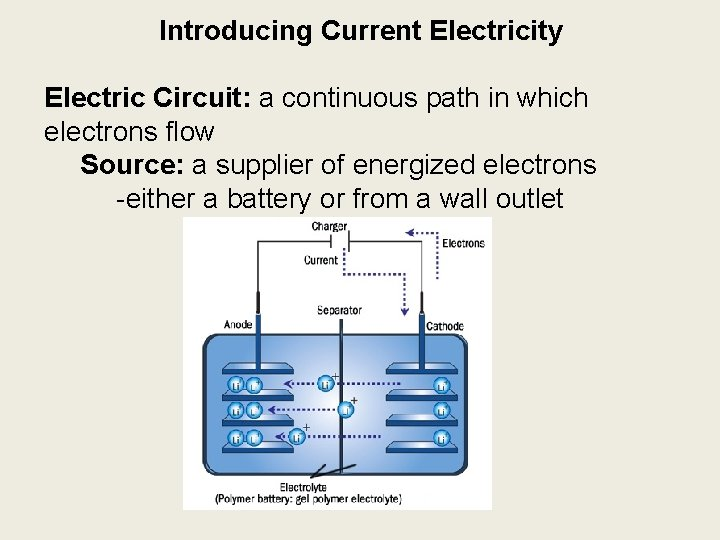Introducing Current Electricity Electric Circuit: a continuous path in which electrons flow Source: a supplier of energized electrons -either a battery or from a wall outlet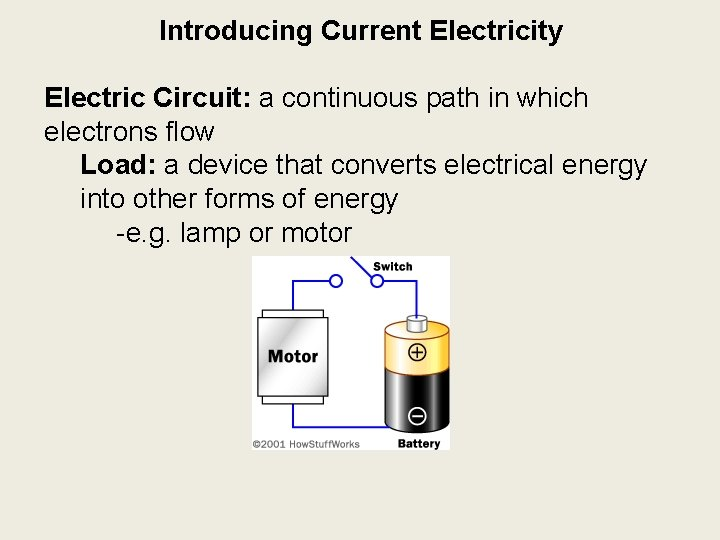Introducing Current Electricity Electric Circuit: a continuous path in which electrons flow Load: a device that converts electrical energy into other forms of energy -e. g. lamp or motorIntroducing Current Electricity Electric Circuit: a continuous path in which electrons flow Conductors: wires that transmit electrons from the source anode (negative terminal) to the load & back to the source cathode (positive terminal)Introducing Current Electricity Electric Circuit: a continuous path in which electrons flow Switch: a device that controls the flow of electrons by opening the circuit open closed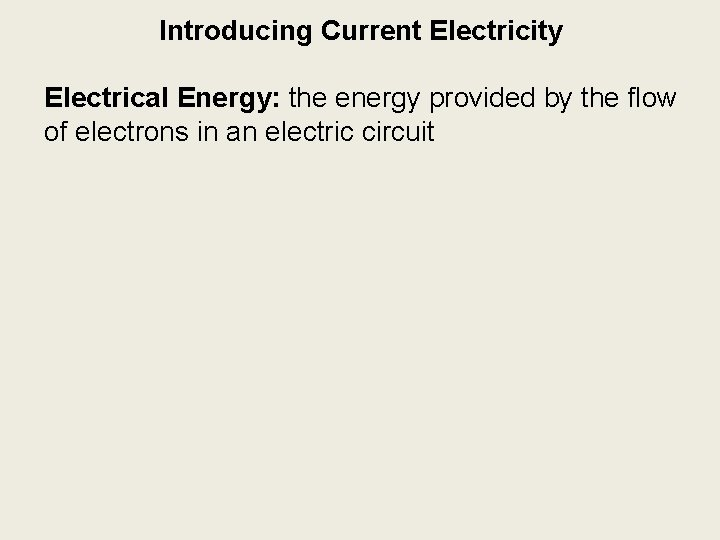Introducing Current Electricity Electrical Energy: the energy provided by the flow of electrons in an electric circuitIntroducing Current Electricity Electrical Energy: the energy provided by the flow of electrons in an electric circuit Electric Cell: a device that converts chemical energy into electrical energy Batteries: can contain one or many cells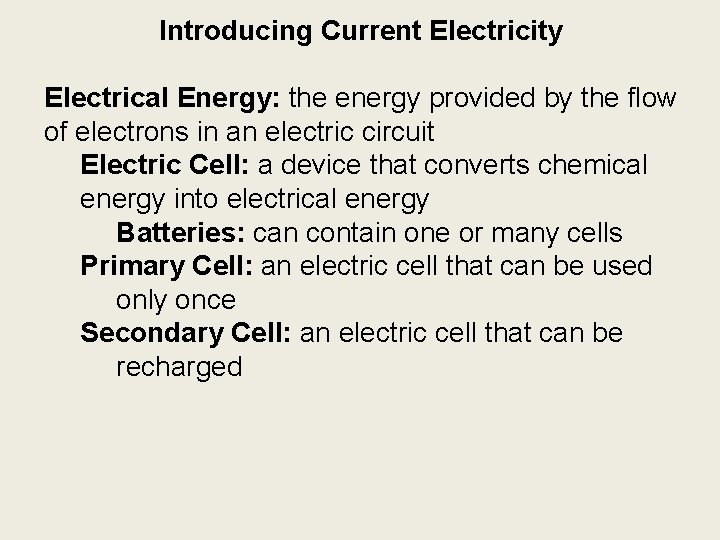Introducing Current Electricity Electrical Energy: the energy provided by the flow of electrons in an electric circuit Electric Cell: a device that converts chemical energy into electrical energy Batteries: can contain one or many cells Primary Cell: an electric cell that can be used only once Secondary Cell: an electric cell that can be recharged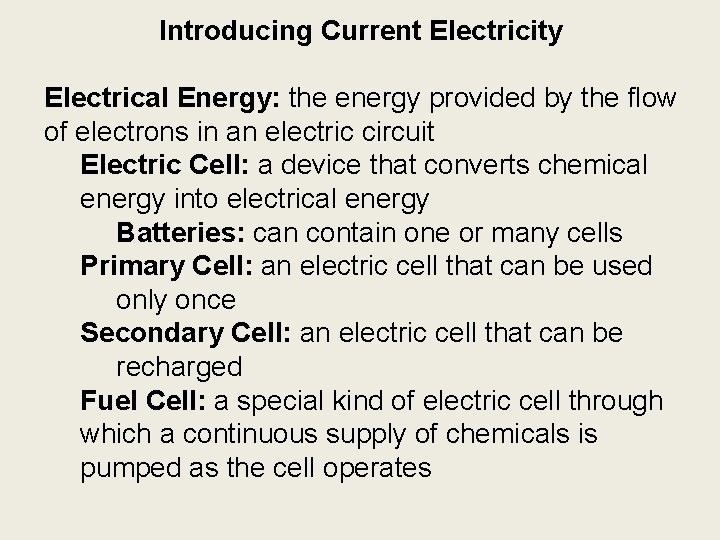Introducing Current Electricity Electrical Energy: the energy provided by the flow of electrons in an electric circuit Electric Cell: a device that converts chemical energy into electrical energy Batteries: can contain one or many cells Primary Cell: an electric cell that can be used only once Secondary Cell: an electric cell that can be recharged Fuel Cell: a special kind of electric cell through which a continuous supply of chemicals is pumped as the cell operatesIntroducing Current Electricity Forms of Current Electricity Direct Current: a flow of electrons in one direction through an electric circuit -form produced by batteries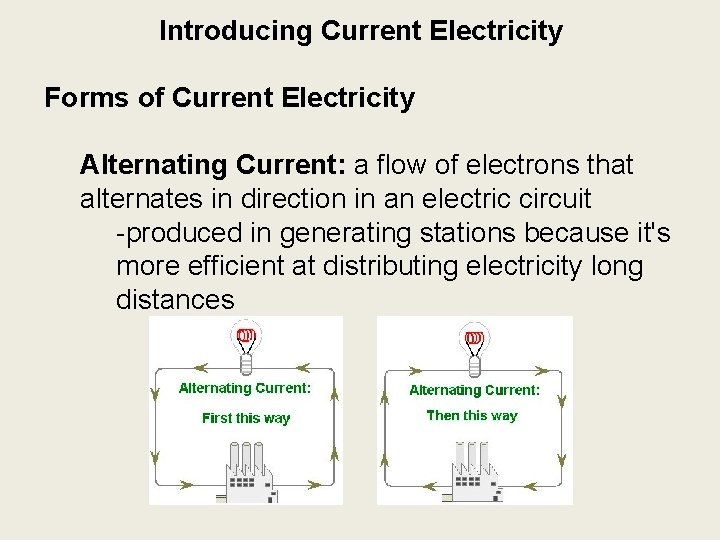Introducing Current Electricity Forms of Current Electricity Alternating Current: a flow of electrons that alternates in direction in an electric circuit -produced in generating stations because it's more efficient at distributing electricity long distances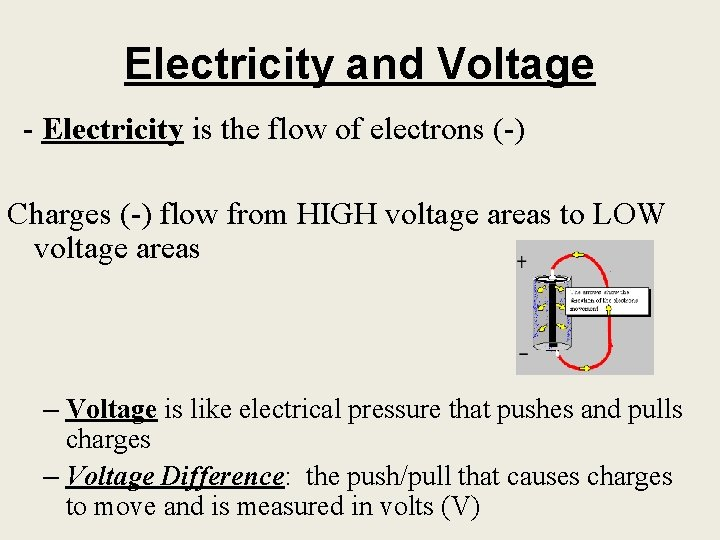Electricity and Voltage - Electricity is the flow of electrons (-) Charges (-) flow from HIGH voltage areas to LOW voltage areas – Voltage is like electrical pressure that pushes and pulls charges – Voltage Difference: the push/pull that causes charges to move and is measured in volts (V)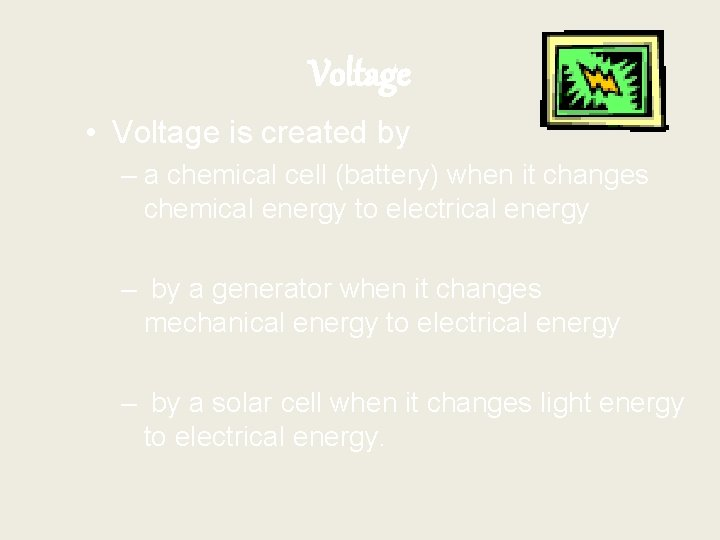Voltage • Voltage is created by – a chemical cell (battery) when it changes chemical energy to electrical energy – by a generator when it changes mechanical energy to electrical energy – by a solar cell when it changes light energy to electrical energy.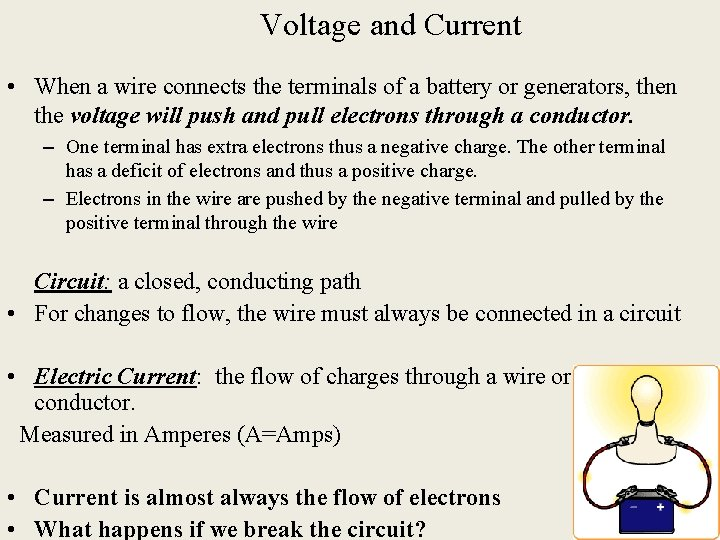Voltage and Current • When a wire connects the terminals of a battery or generators, then the voltage will push and pull electrons through a conductor. – One terminal has extra electrons thus a negative charge. The other terminal has a deficit of electrons and thus a positive charge. – Electrons in the wire are pushed by the negative terminal and pulled by the positive terminal through the wire Circuit: a closed, conducting path • For changes to flow, the wire must always be connected in a circuit • Electric Current: the flow of charges through a wire or any conductor. Measured in Amperes (A=Amps) • Current is almost always the flow of electrons • What happens if we break the circuit?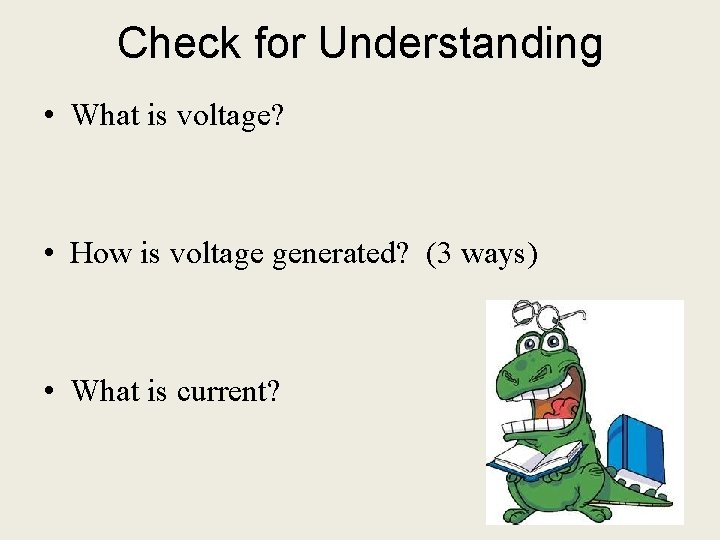Check for Understanding • What is voltage? • How is voltage generated? (3 ways) • What is current?Resistance • Resistance: the tendency for a material to oppose the flow of electrons • Changes electrical energy into thermal energy and light • Ex: lightbulb filament • Resistance is measured in Ohms (Ω)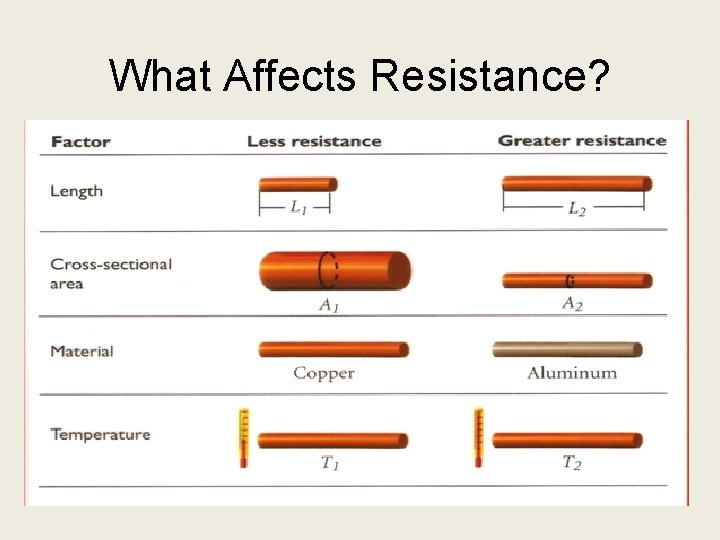What Affects Resistance?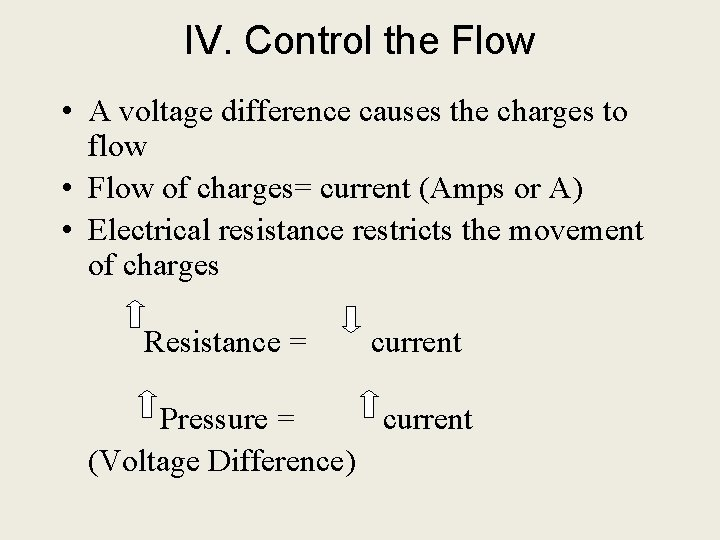IV. Control the Flow • A voltage difference causes the charges to flow • Flow of charges= current (Amps or A) • Electrical resistance restricts the movement of charges Resistance = current Pressure = current (Voltage Difference)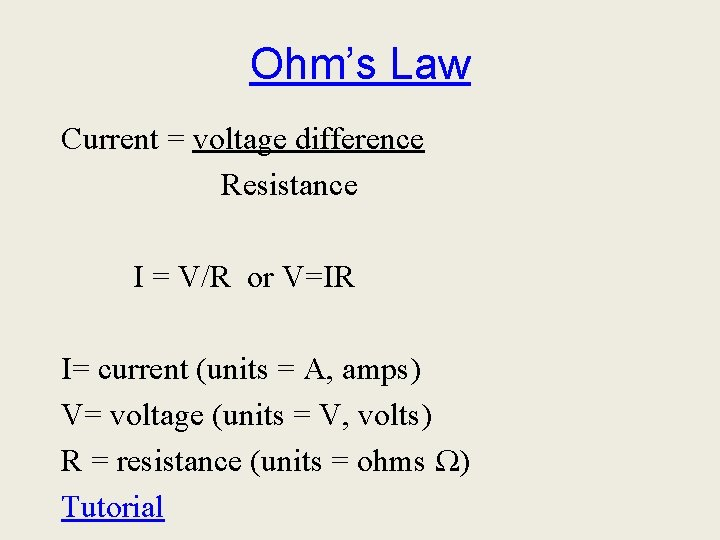Ohm’s Law Current = voltage difference Resistance I = V/R or V=IR I= current (units = A, amps) V= voltage (units = V, volts) R = resistance (units = ohms Ω) Tutorial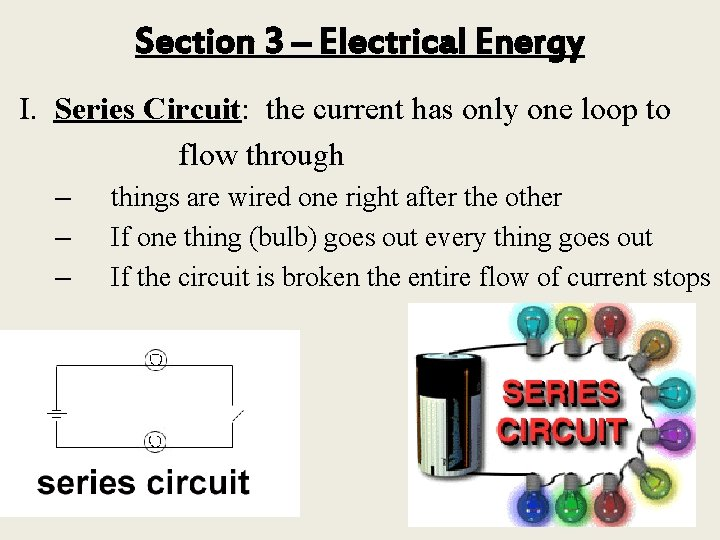Section 3 – Electrical Energy I. Series Circuit: the current has only one loop to flow through – – – things are wired one right after the other If one thing (bulb) goes out every thing goes out If the circuit is broken the entire flow of current stops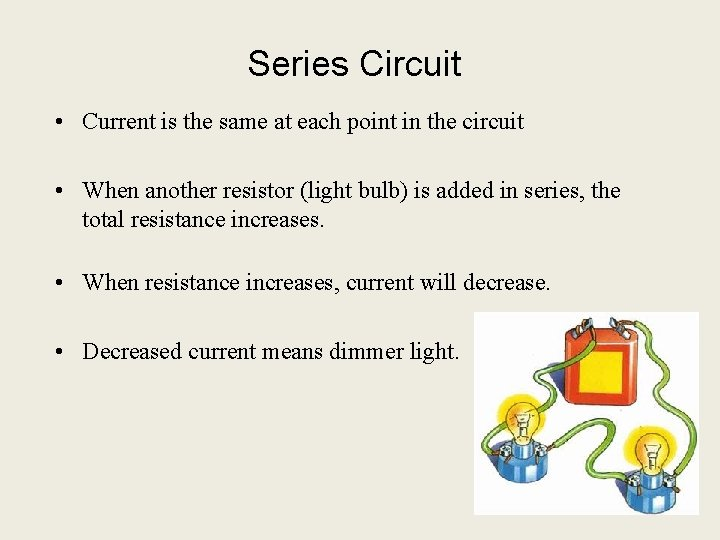Series Circuit • Current is the same at each point in the circuit • When another resistor (light bulb) is added in series, the total resistance increases. • When resistance increases, current will decrease. • Decreased current means dimmer light.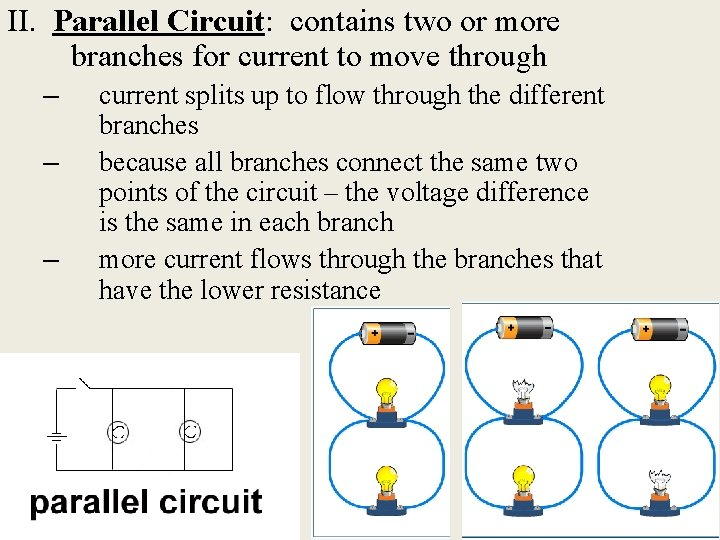II. Parallel Circuit: contains two or more branches for current to move through – – – current splits up to flow through the different branches because all branches connect the same two points of the circuit – the voltage difference is the same in each branch more current flows through the branches that have the lower resistanceHousehold Electrical Safety • In a house, many appliances draw current from the same circuit – If more appliances are connected to a circuit, more current will flow through the wires – More current in wires = more heating in the wires – More heat causes insulation on wires to melt, which increases chances of fire • To protect a house from this, all household circuits have a: 1. Fuse, or 2. Circuit breaker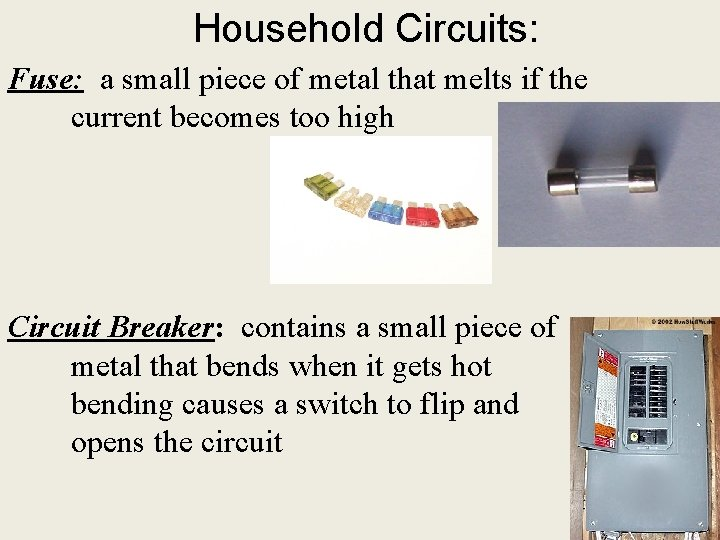Household Circuits: Fuse: a small piece of metal that melts if the current becomes too high Circuit Breaker: contains a small piece of metal that bends when it gets hot bending causes a switch to flip and opens the circuit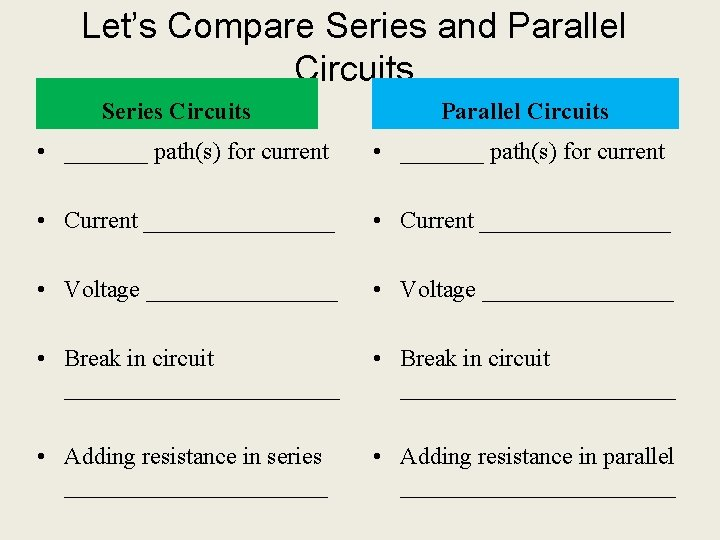Let’s Compare Series and Parallel Circuits Series Circuits Parallel Circuits • _______ path(s) for current • Current ________________ • Voltage ________________ • Break in circuit _______________________ • Adding resistance in series ___________ • Adding resistance in parallel ____________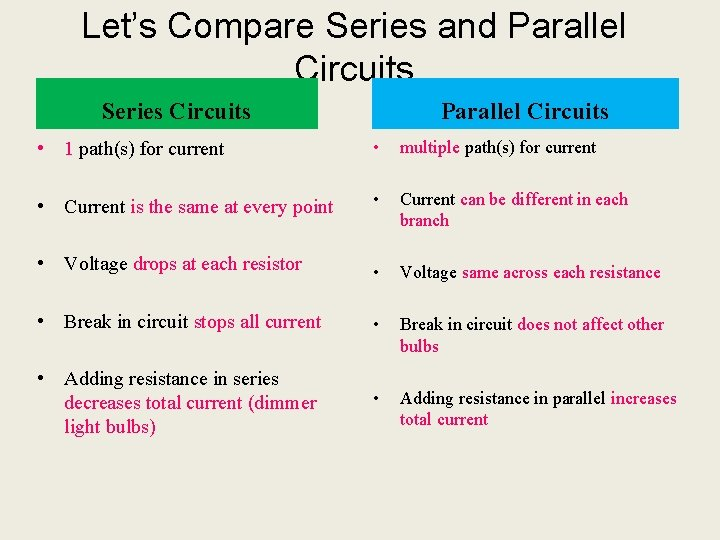Let’s Compare Series and Parallel Circuits Series Circuits Parallel Circuits • 1 path(s) for current • multiple path(s) for current • Current is the same at every point • Current can be different in each branch • Voltage drops at each resistor • Voltage same across each resistance • Break in circuit stops all current • Break in circuit does not affect other bulbs • Adding resistance in series decreases total current (dimmer light bulbs) • Adding resistance in parallel increases total current